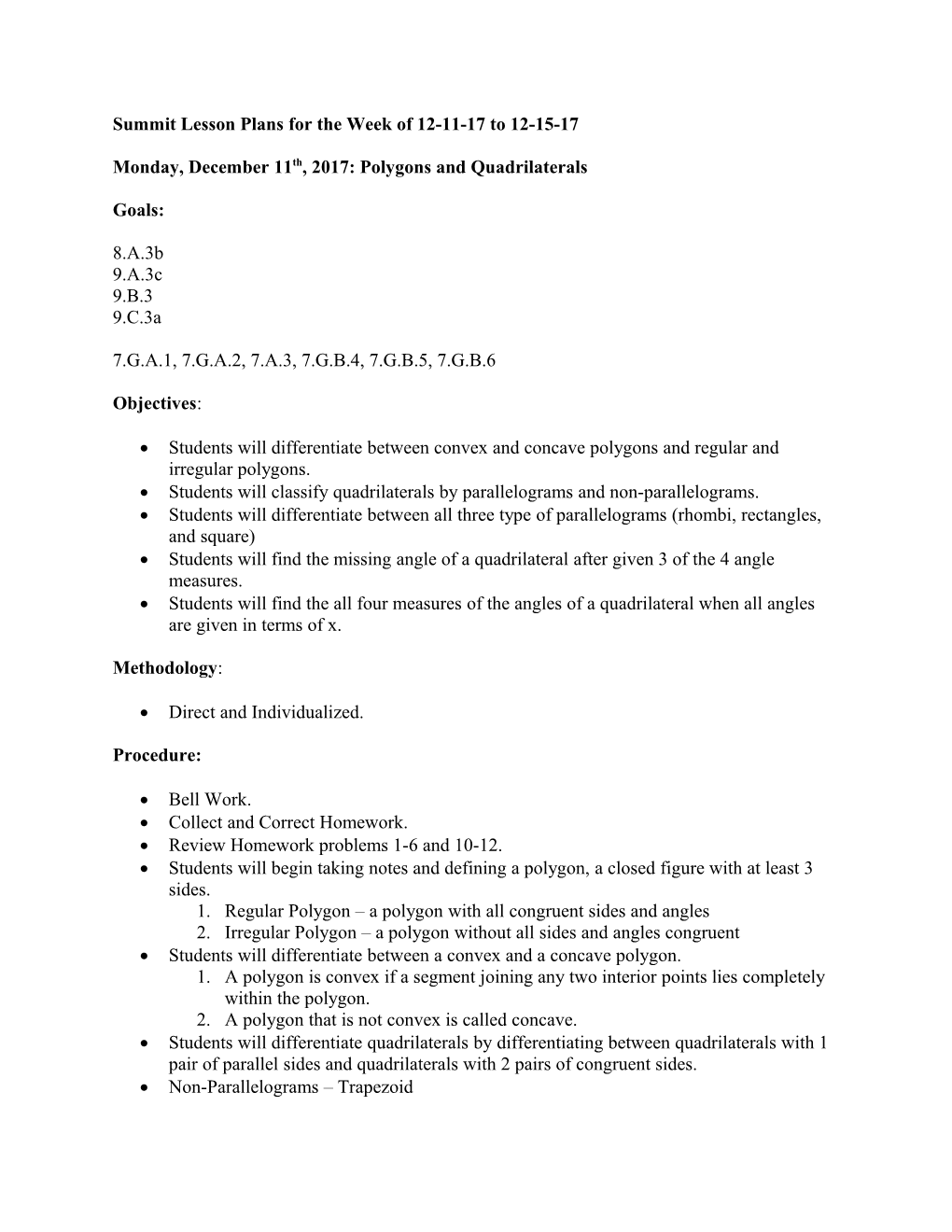# Summit Lesson Plans for the Week of 12-11-17 to 12-15-17Summit Lesson Plans for the Week of 12-11-17 to 12-15-17

Monday, December 11th, 2017: Polygons and Quadrilaterals

Goals:

8.A.3b

9.A.3c

9.B.3

9.C.3a

7.G.A.1, 7.G.A.2, 7.A.3, 7.G.B.4, 7.G.B.5, 7.G.B.6

Objectives:

• Students will differentiate between convex and concave polygons and regular and irregular polygons.
• Students will classify quadrilaterals by parallelograms and non-parallelograms.
• Students will differentiate between all three type of parallelograms (rhombi, rectangles, and square)
• Students will find the missing angle of a quadrilateral after given 3 of the 4 angle measures.
• Students will find the all four measures of the angles of a quadrilateral when all angles are given in terms of x.

Methodology:

• Direct and Individualized.

Procedure:

• Bell Work.
• Collect and Correct Homework.
• Review Homework problems 1-6 and 10-12.
• Students will begin taking notes and defining a polygon, a closed figure with at least 3 sides.
• Regular Polygon – a polygon with all congruent sides and angles
• Irregular Polygon – a polygon without all sides and angles congruent
• Students will differentiate between a convex and a concave polygon.
• A polygon is convex if a segment joining any two interior points lies completely within the polygon.
• A polygon that is not convex is called concave.
• Students will differentiate quadrilaterals by differentiating between quadrilaterals with 1 pair of parallel sides and quadrilaterals with 2 pairs of congruent sides.
• Non-Parallelograms – Trapezoid
• Parallelograms – Rhombus (4 congruent sides), Rectangle (4 right angles, and a square (4 right angles and 4 congruent sides).
• Students will find the missing angle of a quadrilateral after given 3 of the 4 angle measures.
• Students will find the all four measures of the angles of a quadrilateral when all angles are given in terms of x.
• Classwork: Practice 5-3
• Students will begin their homework, Are and Perimeter Packet Page One

Assessment:

• Informal individualized and whole group assessment.
• Homework:

Tuesday, December 12th, 2017: STAR Testing

Wednesday, December 13th, 2017: Area and Perimeter

• Students will use today’s class to work on their Area and Perimeter Packet.
• I will introduce Properties of Circle if time allows.
• Homework: Finish Area and Perimeter Packet.

Thursday, December 14th, 2017: Circumference and Area of Circumference

Goals:

7.G.A.1, 7.G.A.2, 7.A.3, 7.G.B.4, 7.G.B.5, 7.G.B.6

Objectives:

• Students will engage in hands-on activities to find the derivative of the formulas for circumference and area.
• Students will accurately find both the circumference and area of circles.
• Students will find the radius and/or diameter of a circle given its area or circumference.

Methodology:

• Direct and Whole Group Instruction.

Procedure:

• Bell Work Quiz.
• Correct/Collect Homework.
• Circle Notes.
• Hands-On Investigations with Coffee filter
• Guided Practice.
• Homework: 10.4/Practice B

Assessment:

• Informal individualized and whole group assessment.
• Homework: 10.4/Practice B

Friday, December 15th, 2017: Irregular Figures and Intro to Mall Project (if time allows)

Goals:

7.G.A.1, 7.G.A.2, 7.A.3, 7.G.B.4, 7.G.B.5, 7.G.B.6

Objectives:

• Students will decipher how to break irregular figures into known shapes to find the composite area.
• Students will solve real-world problems using their comprehension of composite shapes.

Methodology:

• Direct and Whole Group Instruction.

Procedure:

• Bell Work.
• Collect and Correct Homework.
• Guided Practice: Pg. 277—280: 1-4
• Guided Practice: Pg. 280: 1-4
• Number Talk/Math Challenge Problem
• Homework: Worksheet: Finding the Area of Irregular Figures.

Assessment:

• Informal individualized and whole group assessment.
• Homework: Worksheet: Finding the Area of Irregular Figures.